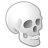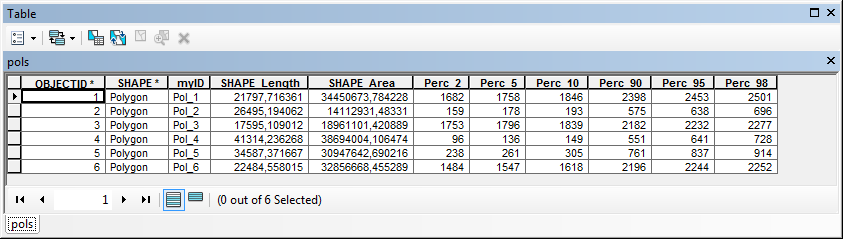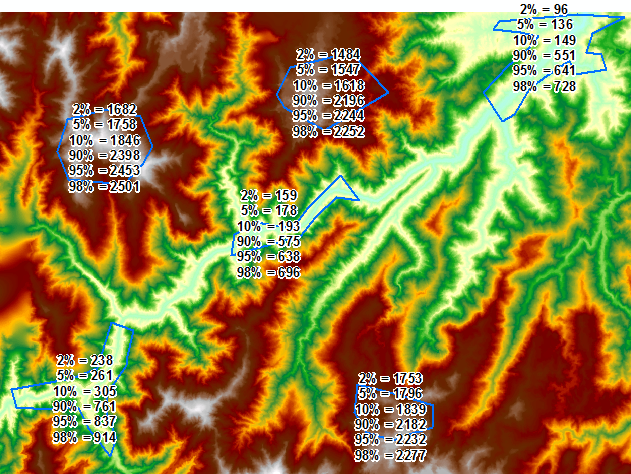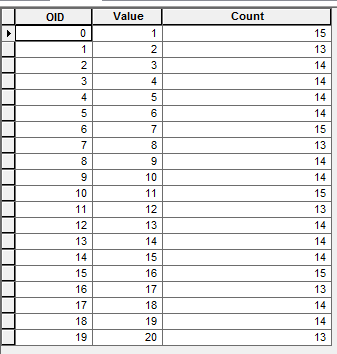# Zonal statistics - percentiles

22065
65
06-30-2014 01:35 PMby
New Contributor III
Hello,

I am looking for an analogous method to Zonal Statistics that is able to generate user-defined percentiles (such as the 5th and 95th percentile) of the input raster data.

I looked at the GME tools and those stats metrics are hardwired in the same fashion as ArcGIS Zonal Statistics tools are.

Any other ideas that I can explore?
Tags (2)
1 Solution

Accepted SolutionsbyEsri Esteemed Contributor

OK, so far for posting code without testing it ...

I created a small test dataset, consisting of a DEM (integer raster) and some random polygons. During the test a number of errors in the code were revealed. These are corrected in the code below:

```def GetPixelValueForPercentile(dctper, percentile):
"""will return last pixel value
where percentile LE searched percentile."""
for k in sorted(dctper.keys()):
perc = dctper
if perc <= percentile:
pix_val = k
else:
break
return pix_val

def FieldExist(featureclass, fieldname):
"""Check if field exists"""
import arcpy
fieldList = arcpy.ListFields(featureclass, fieldname)
return len(fieldList) == 1

import arcpy
# settings
ras = r"D:\Xander\tmp\ras.gdb\DEMsmpro"
fc = r"D:\Xander\tmp\ras.gdb\pols"
# ras = r"C:\Path\To\Folder\Or\Geodatabase\With\Raster"
# fc = r"C:\Path\To\Folder\Or\Geodatabase\With\PolygonFeatureclass"
lst_perc = [2, 5, 10, 90, 95, 98] # list of percentiles to be calculated
fld_prefix = "Perc_"

arcpy.env.workspace = "IN_MEMORY"
arcpy.env.overwriteOutput = True

# add fields if they do not exist in fc
# be aware, field that exist will be overwritten!
print "filling field list and adding fields"
flds = []
for perc in lst_perc:
fld_perc = "{0}{1}".format(fld_prefix, perc)
flds.append(fld_perc)
if not FieldExist(fc, fld_perc):
print "flds={0}".format(flds)

# Enable Spatial analyst
arcpy.CheckOutExtension("Spatial")

# loop through polygons
print "loop through polygons"
flds.append("SHAPE@")
i = 0
with arcpy.da.UpdateCursor(fc, flds) as curs:
for row in curs:
i += 1
print "Processing polygon: {0}".format(i)
polygon = row[flds.index("SHAPE@")]
lst_parts = []
if polygon.partCount == 1:
for part in polygon:
for pnt in part:
x, y = pnt.X, pnt.Y
lst_parts.append(arcpy.Point(x, y))
else:
for part in polygon:
lst_crds = []
for pnt in part:
x, y = pnt.X, pnt.Y
lst_crds.append(arcpy.Point(x, y))
lst_parts.append(lst_crds)
print " - lst_parts={0}".format(lst_parts)

# Execute ExtractByPolygon (you can't send the polygon object)
print " - ExtractByPolygon..."
ras_pol = arcpy.sa.ExtractByPolygon(ras, lst_parts, "INSIDE")
outname = "ras{0}".format(i)
ras_pol.save(outname)
print " - saved raster as {0}".format(outname)

# create dictionary with value vs count
print " - fill dict with Value x Count"
flds_ras = ("VALUE", "COUNT")
dct = {row:row for row in arcpy.da.SearchCursor(outname, flds_ras)}

# calculate number of pixels in raster
print " - determine sum"
cnt_sum = sum(dct.values())
print " - sum={0}".format(cnt_sum)

# loop through dictionary and create new dictionary with val vs percentile
print " - create percentile dict"
dct_per = {}
cnt_i = 0
for val in sorted(dct.keys()):
cnt_i += dct[val]
dct_per[val] = cnt_i / cnt_sum

# loop through list of percentiles
print " - iterate perceniles"
for perc in lst_perc:
# use dct_per to determine percentiles
perc_dec = float(perc) / 100
print "   - Perc_dec for is {0}".format(perc_dec)
pixval =  GetPixelValueForPercentile(dct_per, perc_dec)
print "   - Perc for {0}% is {1}".format(perc, pixval)

# write pixel value to percentile field
print "   - Store value"
fld_perc = "{0}{1}".format(fld_prefix, perc)
row[flds.index(fld_perc)] = pixval

# update row
print " - update row"
curs.updateRow(row)

arcpy.CheckInExtension("Spatial")
```

What you get are a number of fields in your polygon featureclass:When you add some labels to display the values, you'll see that they actually correspond to the areas:So Todd, I hope this will work for you.

Kind regards,

Xander

65 RepliesbyMVP Esteemed Contributor
I am looking for an analogous method to Zonal Statistics that is able to generate user-defined percentiles (such as the 5th and 95th percentile) of the input raster data.

The Slice tool is a handy way to do this. If you specify an EQUAL_AREA slice with 20 zones specified, the first class will be in the 5th percentile, the last will be in the 95th.

If your data values are not continuously distributed (that is, many ties, which can make the percentile value less useful) or highly skewed (which can get you into numerical issues) you may not get the results you expect, but if the data are well-distributed this approach should work very well.by
New Contributor III
Thanks for the Slice suggestion.

I ran the tool using the parameters you suggested. However, the raster attribute table that was returned simply shows 20 rows with "Values" of 1 through 20 and a "Count" of the number of pixels in each of these equal area classes.

I don't see how I can derive the percentile values from this information?byMVP Esteemed Contributor

You then analyze your sliced raster with Zonal Statistics As Table to get the min and max for each class.by
New Contributor III

It may be that my slice raster was not generated properly (attribute table shown below). It looks like it returned the number of cells in each class, which are all about the same since I used equal area classes.

I don't see how to analyze this sliced raster with zonal statistics to generate a table of percentiles?byMVP Esteemed Contributor

I'm sorry Todd - still working on how to track threads in this new system, hence no reply til now.

If you ask for 20 classes from the Slice tool with the equal area option, the first class is the 5th percentile, ie VALUE times 5 (100 / 20).

If you want the percentile values (breaks), you can run Zonal Statistics As Table using the sliced raster for zones and the input to the slice for values.byEsri Esteemed Contributor

Hi Todd,

Maybe a little Python can help: Look at the code below:

```def GetPixelValueForPercentile(dctper, percentile):
"""will return last pixel value
where percentile LE searched percentile."""
for k in sorted(dctper.keys()):
perc = dctper
if perc <= percentile:
pix_val = k
else:
break
return pix_val

import arcpy

# path to raster (has to be integer!)
ras = r"C:\Path\To\Folder\Or\Geodatabase\With\Raster"

# create dictionary with value vs count
flds = ("VALUE", "COUNT")
dct = {row:row for row in arcpy.da.SearchCursor(ras, flds)}

# calculate number of pixels in raster
cnt_sum = sum(dct.values())

dct_per = {}
cnt_i = 0

# loop through dictionary and create new dictionary with val vs percentile
for val in sorted(dct.keys()):
cnt_i += dct[val]
dct_per[val] = cnt_i / cnt_sum

# use dct_per to determine percentiles
perc = 0.05 # (5%)
pixval =  GetPixelValueForPercentile(dct_per, perc)
print "Found for percentile {0}, pixel value {1} with real percentile {2}".format(perc, pixval, dct_per[pixval])

perc = 0.95 # (95%)
pixval =  GetPixelValueForPercentile(dct_per, perc)
print "Found for percentile {0}, pixel value {1} with real percentile {2}".format(perc, pixval, dct_per[pixval])
```

It will return the following information:

```>> Found for percentile 0.05, pixel value 401 with real percentile 0.049861616298
>> Found for percentile 0.95, pixel value 2350 with real percentile 0.949769065993
```

The code requires that your raster is of type integer (to make sure it has an attribute table with pixelvalue and count.

This is what happens in the code:

• on line 16 a path to your integer raster is defined (e.g. ras = r'D:\Xander\tmp\ras.gdb\DEMsmallInt')
• on line 20 the attribute table is used by a da search cursor to create a dictionary (this helps to speed up the proces)
• on line 23 the sum of the count is calculated (the number of pixel with a value in the raster)
• on line 29 a dictionary is created that holds the pixel value and corresponding percentile
• on line 35 and 39 the function (defined at the top) is used to search the pixel value for a percentile value.

Hope this is of any use.

Kind regards, Xanderby
New Contributor III

Thanks for the Python code for percentiles from a raster Xander.

In my situation, I have multiple polygons that occur in various locations across a raster. I would like to generate the percentile statistics from the various portions of the raster that overlap with each individual polygon. Then, join those statistics with a unique row ID in the polygon layer.

I am essentially in need of "Zonal Statistics as Table" with the ability to include percentile statistics along with Minimum, Mean, and Maximum.

How can this be incorporated via Python?byEsri Esteemed Contributor

Hi Todd,

One option is that you write a loop through the polygon (Update cursor) to do this for each polygon:

• use the arcpy.sa.ExtractByPolygon tool to extract the relevant portion of raster and save that to the IN_MEMORY workspace
• use that raster and the previous posted code to determine the percentiles
• define a list of percentile values like lst_prec = [2, 5,10, 90, 95, 98] to loop through the values and determine the raster value that corresponds to that percentile. Store the result in previously created fields.

In case there is no overlap between the polygons, you could do the following:

• Use the arcpy.sa.Combine tool to create a raster that holds the combination of both values. If the number of unique combinations is less than 65536, this should be no problem.
• Adapt the code to read both the polygon layer in an update cursor and the combine raster attribute table in a search cursor to determine the percentiles and store the result

Not sure which option will be the fastest.

Now to throw in some code (which by the way I did not test), it should look something like this:

```def GetPixelValueForPercentile(dctper, percentile):
"""will return last pixel value
where percentile LE searched percentile."""
for k in sorted(dctper.keys()):
perc = dctper
if perc <= percentile:
pix_val = k
else:
break
return pix_val

def FieldExist(featureclass, fieldname):
"""Check if field exists"""
import arcpy
fieldList = arcpy.ListFields(featureclass, fieldname)
return len(fieldList) == 1

import arcpy
# settings
ras = r"C:\Path\To\Folder\Or\Geodatabase\With\Raster"
fc = r"C:\Path\To\Folder\Or\Geodatabase\With\PolygonFeatureclass"
lst_perc = [2, 5, 10, 90, 95, 98] # list of percentiles to be calculated
fld_prefix = "Perc_"

arcpy.env.workspace = "IN_MEMORY"

# add fields if they do not exist in fc
# be aware, field that exist will be overwritten!
flds = []
for perc in lst_perc:
fld_perc = "{0}{1}".format(fld_prefix, perc)
flds.append(fld_perc)
if not FieldExist(fc, fld_perc):

# Enable Spatial analyst
arcpy.CheckOutExtension("Spatial")

# loop through polygons
flds.append("SHAPE@")
with arcpy.da.UpdateCursor(fc, flds) as curs:
for row in curs:
polygon = row[flds.index("SHAPE@")]
lst_parts = []
for part in polygon:
lst_crds = []
for crdpair in part:
x, y = crdpair, crdpair
lst_crds.append(arcpy.Point(x, y))
lst_parts.append(lst_crds)

# Execute ExtractByPolygon
ras_pol = arcpy.sa.ExtractByPolygon(ras, polygon, "INSIDE")
# not sure if ras_pol should be saved...

# create dictionary with value vs count
flds_ras = ("VALUE", "COUNT")
dct = {row:row for row in arcpy.da.SearchCursor(ras_pol, flds_ras)}

# calculate number of pixels in raster
cnt_sum = sum(dct.values())

# loop through dictionary and create new dictionary with val vs percentile
dct_per = {}
cnt_i = 0
for val in sorted(dct.keys()):
cnt_i += dct[val]
dct_per[val] = cnt_i / cnt_sum

# loop through list of percentiles
for perc in lst_perc:
# use dct_per to determine percentiles
prec_dec = float(perc) / 100
pixval =  GetPixelValueForPercentile(dct_per, perc)

# write pixel value to percentile field
fld_perc = "{0}{1}".format(fld_prefix, perc)
row[flds.index(fld_perc)] = pixval

# update row
curs.updateRow(row)

arcpy.CheckInExtension("Spatial")
```

If you run into problems, let me know. Maybe you can throw in a piece of your data to test the code.

Kind regards,

XanderbyEsri Esteemed Contributor

OK, so far for posting code without testing it ...

I created a small test dataset, consisting of a DEM (integer raster) and some random polygons. During the test a number of errors in the code were revealed. These are corrected in the code below:

```def GetPixelValueForPercentile(dctper, percentile):
"""will return last pixel value
where percentile LE searched percentile."""
for k in sorted(dctper.keys()):
perc = dctper
if perc <= percentile:
pix_val = k
else:
break
return pix_val

def FieldExist(featureclass, fieldname):
"""Check if field exists"""
import arcpy
fieldList = arcpy.ListFields(featureclass, fieldname)
return len(fieldList) == 1

import arcpy
# settings
ras = r"D:\Xander\tmp\ras.gdb\DEMsmpro"
fc = r"D:\Xander\tmp\ras.gdb\pols"
# ras = r"C:\Path\To\Folder\Or\Geodatabase\With\Raster"
# fc = r"C:\Path\To\Folder\Or\Geodatabase\With\PolygonFeatureclass"
lst_perc = [2, 5, 10, 90, 95, 98] # list of percentiles to be calculated
fld_prefix = "Perc_"

arcpy.env.workspace = "IN_MEMORY"
arcpy.env.overwriteOutput = True

# add fields if they do not exist in fc
# be aware, field that exist will be overwritten!
print "filling field list and adding fields"
flds = []
for perc in lst_perc:
fld_perc = "{0}{1}".format(fld_prefix, perc)
flds.append(fld_perc)
if not FieldExist(fc, fld_perc):
print "flds={0}".format(flds)

# Enable Spatial analyst
arcpy.CheckOutExtension("Spatial")

# loop through polygons
print "loop through polygons"
flds.append("SHAPE@")
i = 0
with arcpy.da.UpdateCursor(fc, flds) as curs:
for row in curs:
i += 1
print "Processing polygon: {0}".format(i)
polygon = row[flds.index("SHAPE@")]
lst_parts = []
if polygon.partCount == 1:
for part in polygon:
for pnt in part:
x, y = pnt.X, pnt.Y
lst_parts.append(arcpy.Point(x, y))
else:
for part in polygon:
lst_crds = []
for pnt in part:
x, y = pnt.X, pnt.Y
lst_crds.append(arcpy.Point(x, y))
lst_parts.append(lst_crds)
print " - lst_parts={0}".format(lst_parts)

# Execute ExtractByPolygon (you can't send the polygon object)
print " - ExtractByPolygon..."
ras_pol = arcpy.sa.ExtractByPolygon(ras, lst_parts, "INSIDE")
outname = "ras{0}".format(i)
ras_pol.save(outname)
print " - saved raster as {0}".format(outname)

# create dictionary with value vs count
print " - fill dict with Value x Count"
flds_ras = ("VALUE", "COUNT")
dct = {row:row for row in arcpy.da.SearchCursor(outname, flds_ras)}

# calculate number of pixels in raster
print " - determine sum"
cnt_sum = sum(dct.values())
print " - sum={0}".format(cnt_sum)

# loop through dictionary and create new dictionary with val vs percentile
print " - create percentile dict"
dct_per = {}
cnt_i = 0
for val in sorted(dct.keys()):
cnt_i += dct[val]
dct_per[val] = cnt_i / cnt_sum

# loop through list of percentiles
print " - iterate perceniles"
for perc in lst_perc:
# use dct_per to determine percentiles
perc_dec = float(perc) / 100
print "   - Perc_dec for is {0}".format(perc_dec)
pixval =  GetPixelValueForPercentile(dct_per, perc_dec)
print "   - Perc for {0}% is {1}".format(perc, pixval)

# write pixel value to percentile field
print "   - Store value"
fld_perc = "{0}{1}".format(fld_prefix, perc)
row[flds.index(fld_perc)] = pixval

# update row
print " - update row"
curs.updateRow(row)

arcpy.CheckInExtension("Spatial")
```

What you get are a number of fields in your polygon featureclass:When you add some labels to display the values, you'll see that they actually correspond to the areas:So Todd, I hope this will work for you.

Kind regards,

Xander